GFG App
Open AppBrowser
Continue

# Puzzle 29 | (Car Wheel Puzzle)

Puzzle: A car has 4 tyres and 1 spare tyre. Each tyre can travel a maximum distance of 20000 miles before wearing off. What is the maximum distance the car can travel before you are forced to buy a new tyre? You are allowed to change tyres (using the spare tyre) an unlimited number of times.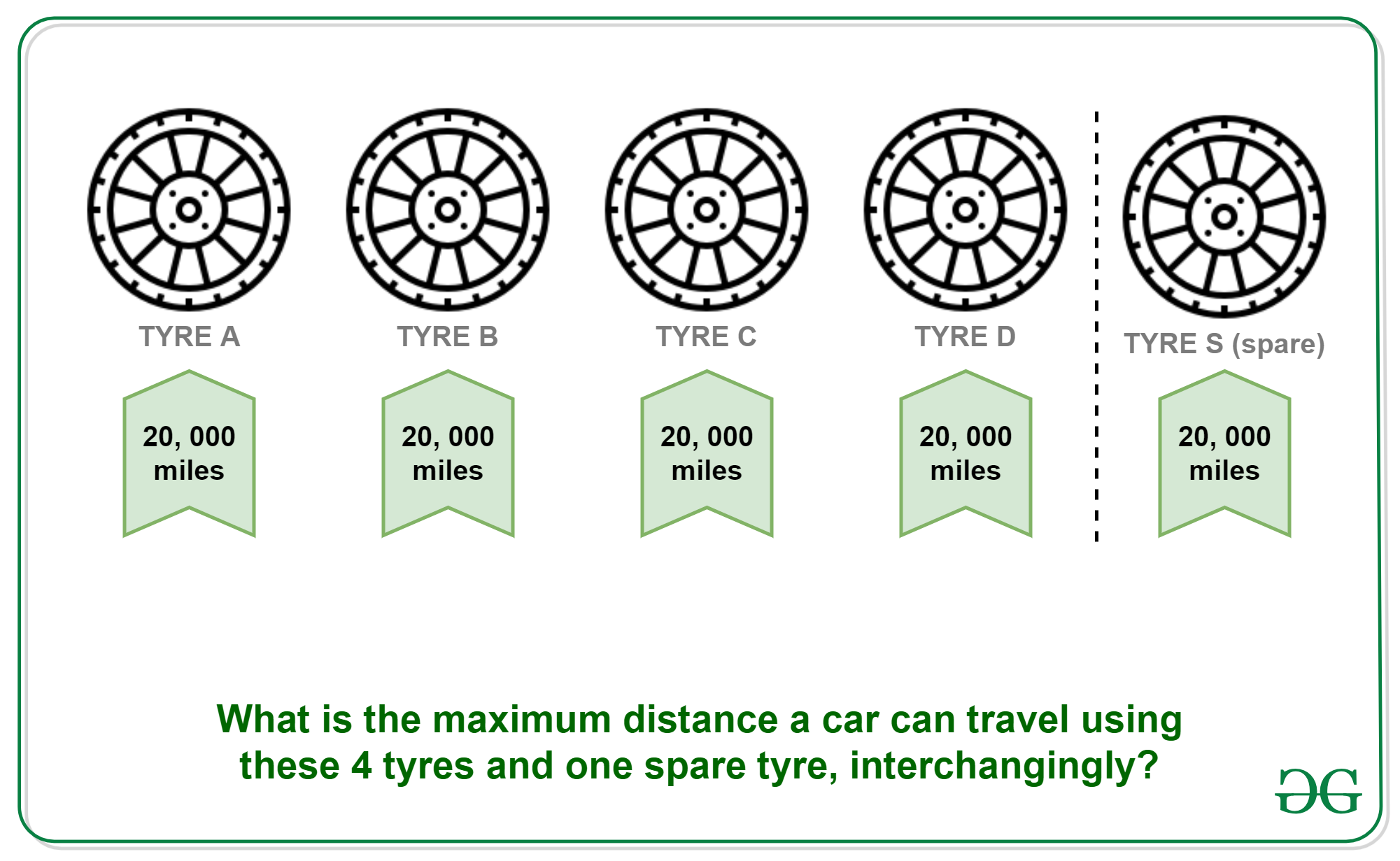Solution: Divide the lifetime of the spare tyre into 4 equal part i.e., 5000 and swap it at each completion of 5000 miles distance.
Let four tyres be named as A, B, C and D and spare tyre be S.

• 5000 KMs: Replace A with S. Remaining distances (A, B, C, D, S) : 15000, 15000, 15000, 15000, 20000.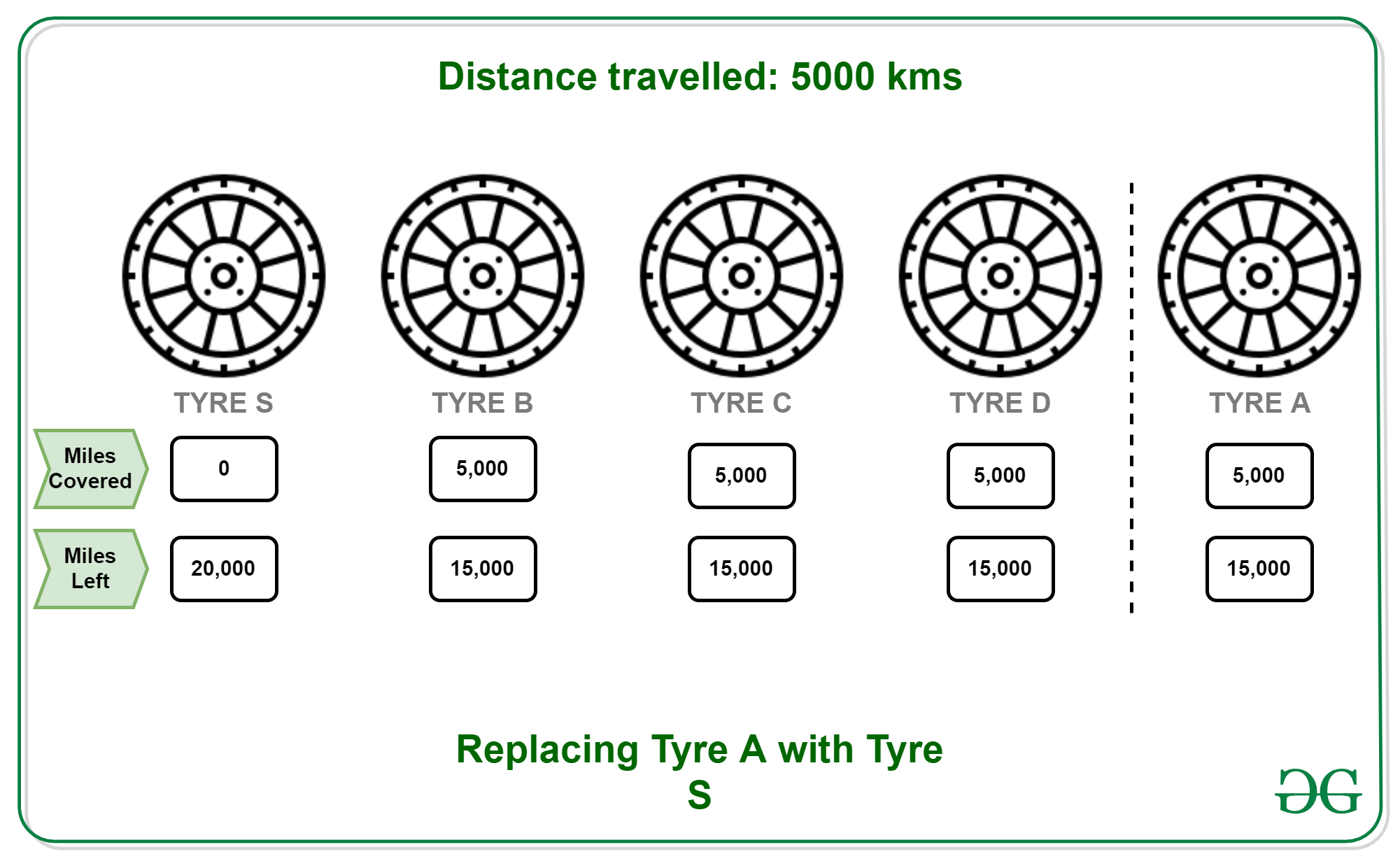• 10000 KMs: Put A back to its original position and replace B with S. Remaining distances (A, B, C, D, S) : 15000, 10000, 10000, 10000, 15000.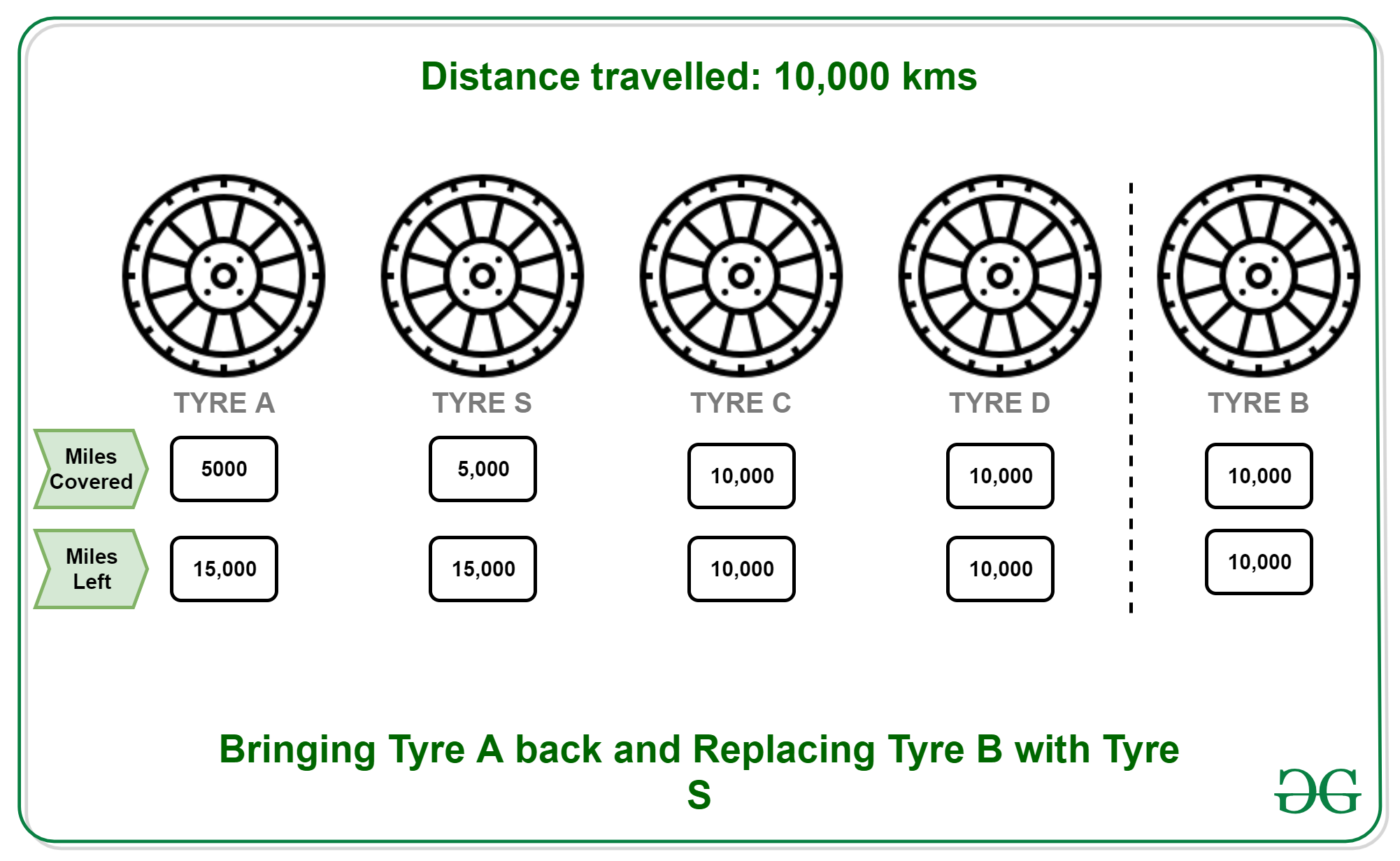• 15000 KMs: Put B back to its original position and replace C with S. Remaining distances (A, B, C, D, S) : 10000, 10000, 5000, 5000, 10000.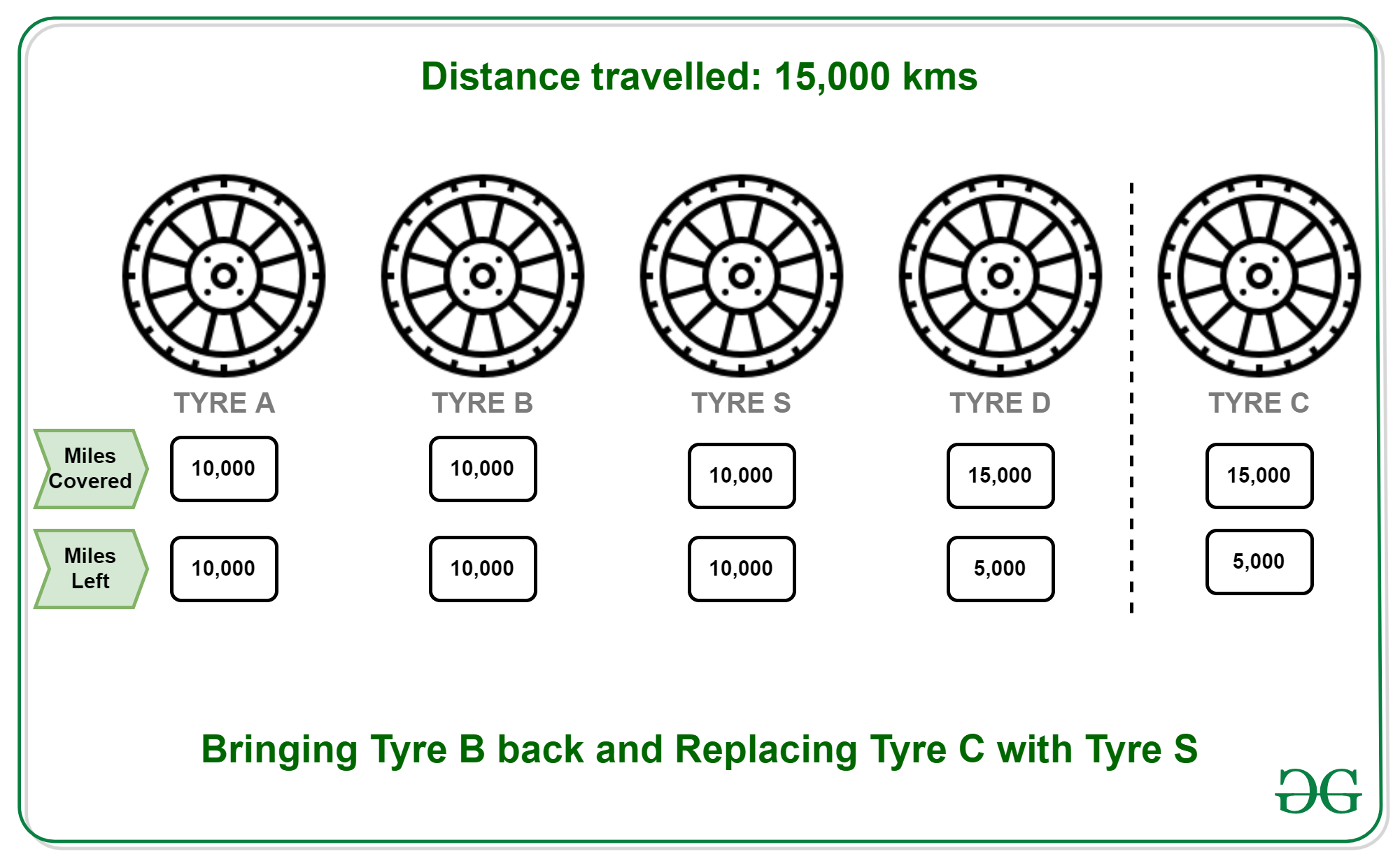• 20000 KMs: Put C back to its original position and replace D with S. Remaining distances (A, B, C, D, S) : 5000, 5000, 5000, 0, 5000.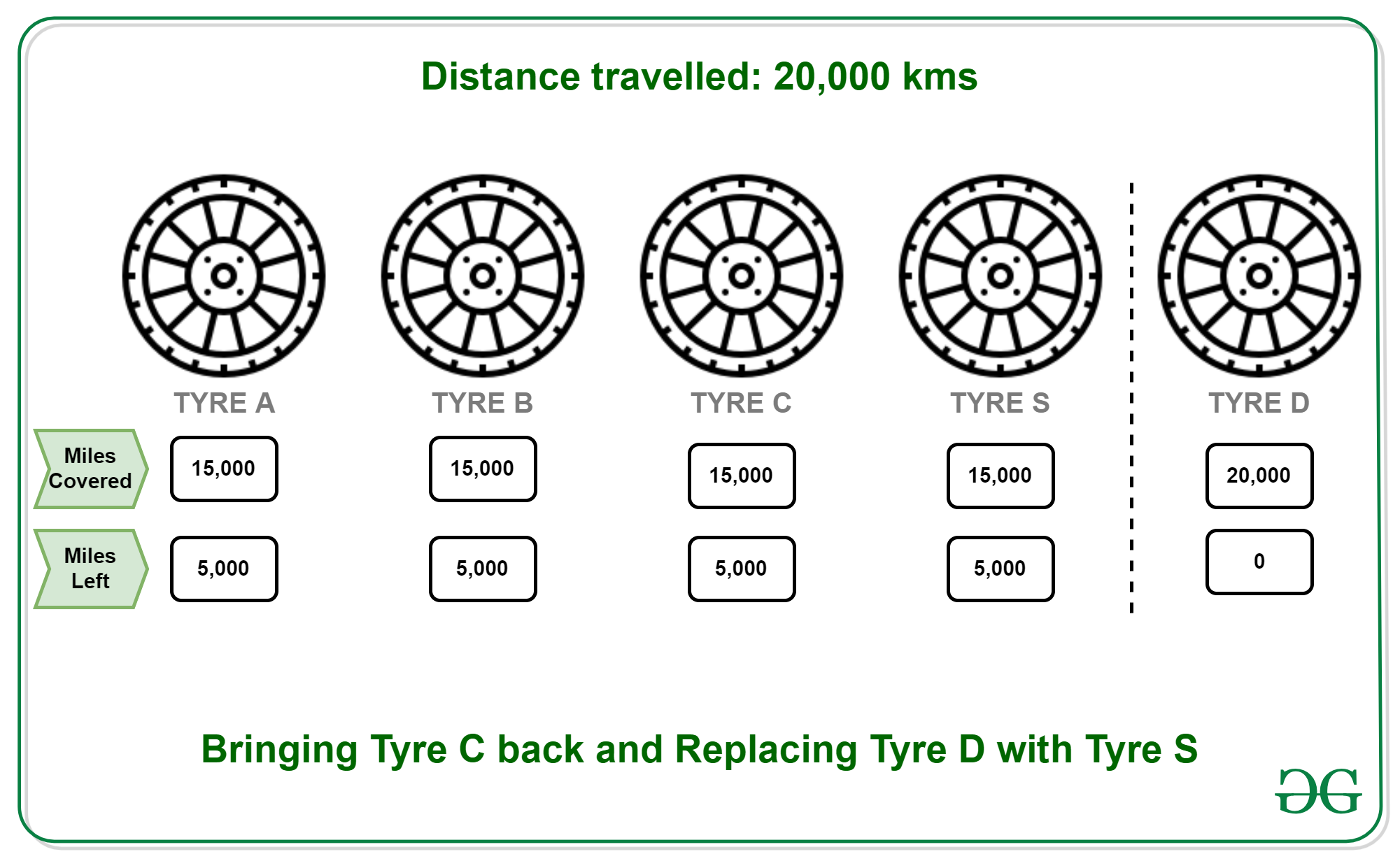• 25000 KMs: Every tyre is now worn out completely.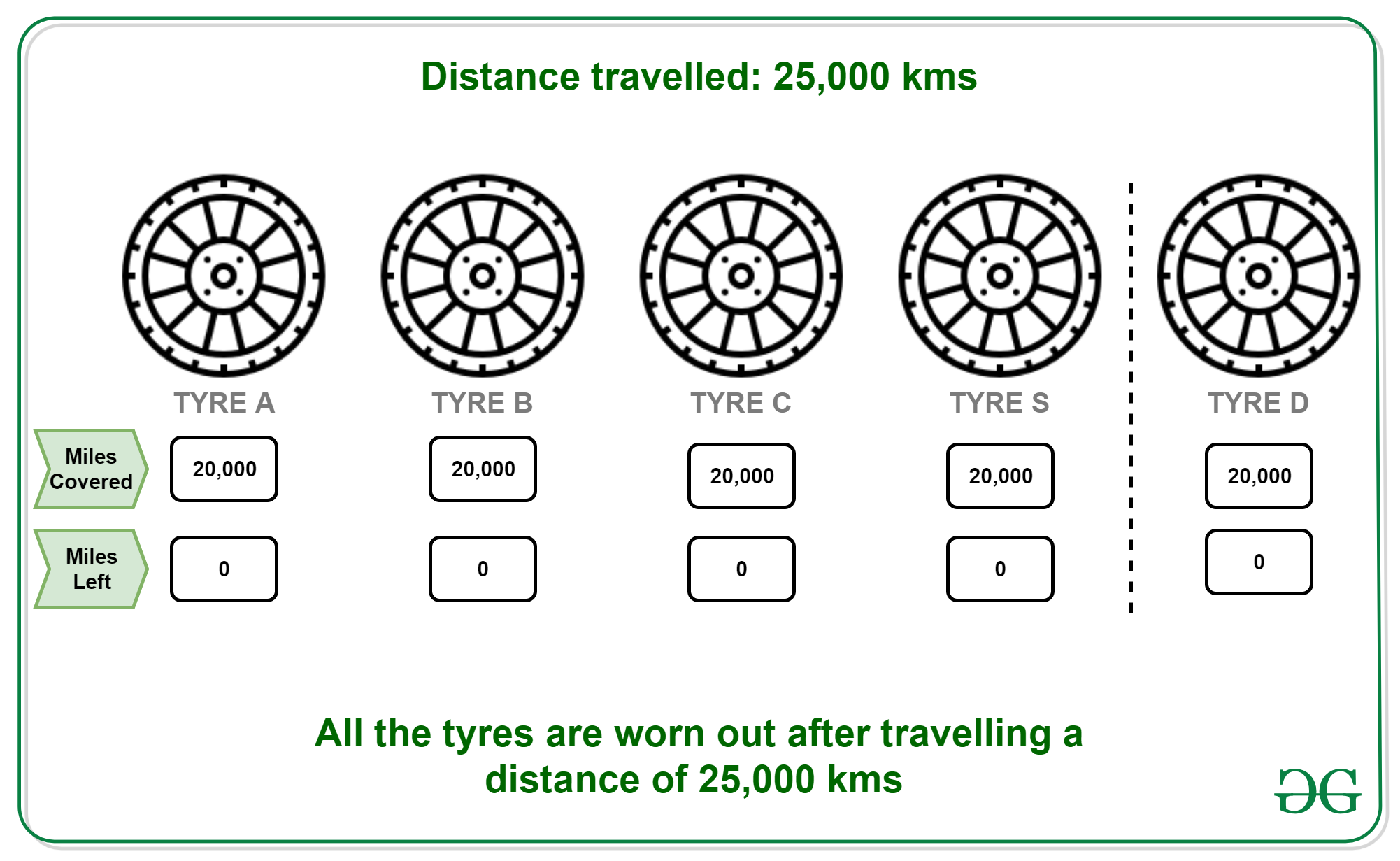All tyres are used to their full strength.

### Related Amazon interview question

There are n pencils, each having l length. Each can write 4 kilometres. After writing 4 kilometers it has l/4 length. One can join 4 pencils which are having l/4 length and can make 1 pencil. One can’t make pencil of pieces if remaining pieces are 3 or 2 or 1 in number but one can include these remaining pieces whenever needed.
Write a relation independent of l, length of the given pencil, for how much one can write from n pencils.
Examples:

```Input: 4
Output: 20```

Recursive Approach:
Suppose we use 3 pencils that will 12 and generate 3 used pencils, now if the remaining pencils are greater than zero at least 1 unused pencil can be used with those 3 unused to write 4 and that will generate 1 more unused pencil. This will keep repeating.

```if(n-3 >= 1){
f(n) = f(n-3) + 12 + 4
}
else{
// Used pencil that cannot be used
f(n) = 4*n
}```

Below is the implementation of the above approach:

## C

 `int` `count(``int` `n) ` `{ ` `    ``if` `(n < 4) { ` `        ``return` `(n * 4); ` `    ``} ` `    ``else` `{ ` `        ``return` `(16 + count(n - 3)); ` `    ``} ` `} `

## Java

 `static` `int` `count(``int` `n) ` `{ ` `    ``if` `(n < ``4``) { ` `        ``return` `(n * ``4``); ` `    ``} ` `    ``else` `{ ` `        ``return` `(``16` `+ count(n - ``3``)); ` `    ``} ` `} ` ` `  `// This code is contributed by Abhijeet Kumar(abhijeet19403)`

## Python3

 `  `  `def` `count( n): ` `    ``if` `(n < ``4``): ` `        ``return` `(n ``*` `4``) ` `    ``else``: ` `        ``return` `(``16` `+` `count(n ``-` `3``)) ` ` `  `# This code is contributed by Abhijeet Kumar(abhijeet19403)`

## C#

 `static` `int` `count(``int` `n) ` `{ ` `    ``if` `(n < 4) { ` `        ``return` `(n * 4); ` `    ``} ` `    ``else` `{ ` `        ``return` `(16 + count(n - 3)); ` `    ``} ` `} ` ` `  `// This code is contributed by Abhijeet Kumar(abhijeet19403)`

Mathematical Approach O(1):
Above relation can be optimized in O(1)

```// x is max no of time we can
//subtract 3 without n-3 <= 3
n - 3*x <= 3
x  >  (n-3)/3
i.e. n/3 - 1 if it divides exactly
else n/3```

Below is the implementation of the above approach:

## CPP

 `// C++ program to find relation independent of l ` `// length of the given pencil, for how much one ` `// can write from n pencils. ` ` `  `#include ` `using` `namespace` `std; ` ` `  `// Function to find no of pencils ` `int` `count(``int` `n) ` `{ ` `    ``int` `x = (n / 3) - 1; ` `    ``if` `(n % 3) { ` `        ``x++; ` `    ``} ` `    ``return` `(4 * x + 4 * n); ` `} ` ` `  `// Driver function ` `int` `main() ` `{ ` `    ``int` `n = 5; ` `    ``cout << count(n) << endl; ` `} `

## Java

 `// Java program to find relation independent of l ` `// length of the given pencil, for how much one ` `// can write from n pencils. ` `class` `GFG ` `{ ` ` `  `// Function to find no of pencils ` `static` `int` `count(``int` `n) ` `{ ` `    ``int` `x = (n / ``3``) - ``1``; ` `    ``if` `(n % ``3` `> ``0``)  ` `    ``{ ` `        ``x++; ` `    ``} ` `    ``return` `(``4` `* x + ``4` `* n); ` `} ` ` `  `// Driver code ` `public` `static` `void` `main(String[] args) ` `{ ` `    ``int` `n = ``5``; ` `    ``System.out.print(count(n) +``"\n"``); ` `} ` `} ` ` `  `// This code is contributed by PrinciRaj1992 `

## Python3

 `# Python3 program to find relation independent of l ` `# length of the given pencil, for how much one ` `# can write from n pencils. ` ` `  `# Function to find no of pencils ` `def` `count(n): ` `    ``x ``=` `(n ``/``/` `3``) ``-` `1``; ` `    ``if` `(n ``%` `3` `> ``0``): ` `        ``x``+``=``1``; ` `     `  `    ``return` `(``4` `*` `x ``+` `4` `*` `n); ` ` `  `# Driver code ` `if` `__name__ ``=``=` `'__main__'``: ` `    ``n ``=` `5``; ` `    ``print``(count(n)); ` `     `  `# This code is contributed by PrinciRaj1992 `

## C#

 `// C# program to find relation independent of l ` `// length of the given pencil, for how much one ` `// can write from n pencils. ` `using` `System; ` ` `  `class` `GFG ` `{ ` ` `  `// Function to find no of pencils ` `static` `int` `count(``int` `n) ` `{ ` `    ``int` `x = (n / 3) - 1; ` `    ``if` `(n % 3 > 0)  ` `    ``{ ` `        ``x++; ` `    ``} ` `    ``return` `(4 * x + 4 * n); ` `} ` ` `  `// Driver code ` `public` `static` `void` `Main(String[] args) ` `{ ` `    ``int` `n = 5; ` `    ``Console.Write(count(n) +``"\n"``); ` `} ` `} ` ` `  `// This code is contributed by 29AjayKumar `

## Javascript

 ` `

Output:

`24`

My Personal Notes arrow_drop_up